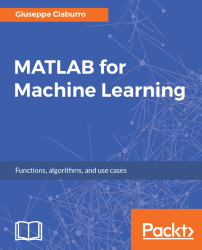•#### MATLAB for Machine Learning#### Overview of this book

MATLAB is the language of choice for many researchers and mathematics experts for machine learning. This book will help you build a foundation in machine learning using MATLAB for beginners. You’ll start by getting your system ready with t he MATLAB environment for machine learning and you’ll see how to easily interact with the Matlab workspace. We’ll then move on to data cleansing, mining and analyzing various data types in machine learning and you’ll see how to display data values on a plot. Next, you’ll get to know about the different types of regression techniques and how to apply them to your data using the MATLAB functions. You’ll understand the basic concepts of neural networks and perform data fitting, pattern recognition, and clustering analysis. Finally, you’ll explore feature selection and extraction techniques for dimensionality reduction for performance improvement. At the end of the book, you will learn to put it all together into real-world cases covering major machine learning algorithms and be comfortable in performing machine learning with MATLAB.
Title PageCreditsForewordwww.PacktPub.comCustomer FeedbackPrefaceFree Chapter
Getting Started with MATLAB Machine LearningImporting and Organizing Data in MATLABFrom Data to Knowledge DiscoveryFinding Relationships between Variables - Regression TechniquesPattern Recognition through Classification AlgorithmsIdentifying Groups of Data Using Clustering MethodsSimulation of Human Thinking - Artificial Neural NetworksImproving the Performance of the Machine Learning Model - Dimensionality ReductionMachine Learning in Practice## Distinguishing the types of variables

Data can be collected in a number of ways; however, the typology of the result can easily be identified by a simple test. If we have to measure a quantity related to a specific event, we collect numbers that identify quantitative variables. If we have to describe the quality of an observed phenomenon, we cannot measure it and we are collecting qualitative variables. Let's understand each one in detail.

### Quantitative variables

Quantitative variables (also called continuous variables) are an expression of a measure and are presented in the form of numerical data. Some examples of quantitative variables are temperature, pressure, and humidity values of a precise location. Quantitative variables can be further categorized as either interval or ratio variables.

Interval variables are variables that assume numeric values ​​that allow comparisons only by difference. It follows that it is possible to order statistical units based on the answers and also to measure...